# Glass

At the glass shop we have to cut 8 sheets of glass. Each was shaped a square with sides of 18 cm. We paid 44 CZK. How much is a 1 m2 of glass?

x =  169.75 Kc

### Step-by-step explanation: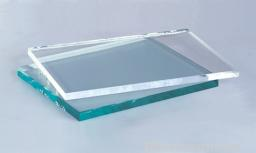Did you find an error or inaccuracy? Feel free to write us. Thank you!Tips to related online calculators
Check out our ratio calculator.

#### You need to know the following knowledge to solve this word math problem:

We encourage you to watch this tutorial video on this math problem:

## Related math problems and questions:

• Glass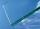Peter broke the window glass with size 110 cm and 90 cm. 1 square meter of glass costs 11 USD. How much money is need to pay for a new glass?
• The kitchenThe kitchen has the shape of a square with a side of 5 m. We place linoleum on the floor, the price of which is 450 CZK per 1 m2. The glue costs 750 CZK. How much do we pay in total?
• Eight masonsEight masons will plaster a wall with an area of 1440 m2 in 9 days. They work 8 hours a day. How much area will plaster 6 masons in 4 hours?
• One deciWine 0,375 l costs 2040 CZK. How much does one dl cost?
• AreaCalculate: x = 1 m2 + 1 dm2 + 1 cm2
• Rails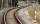18 m railway weighs 1260 kg. How much weighs 100 m of welded railways?
• Snack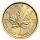CZK 450 was paid for a snack for 30 pupils. How many CZK will we pay for the same snack for 28 pupils?
• PresentGift box has a rectangular shape with dimensions of 8×8×3 cm. Miloslav wants to cover with square paper with sides of 18 cm. How much paper left him?
• Johnny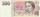Johnny bought for 28 CZK flower to mum. He that spent 4/7 of the money saved. How much money he had saved? How much money left him?
• Concrete boxThe concrete box with walls thick 7 cm has the following external dimensions: length 1.8 m, width 44 cm and height 46 cm. How many liters of soil can fit if I fill it to the brim?
• How muchHow much money will we pay for 20 planks 4m long, 15cm wide and 26mm thick when 1m³ of wood costs 4500kč?
• Two brothersThe two brothers were to be divided according to the will of land at an area of 1ha 86a 30m2 in a ratio of 5:4. How many will everyone get?
• Moneys in triple ratioMilan, John and Lili have a total 344 euros. Their amounts are in the ratio 1:2:5. Determine how much each of them has?
• Bathroom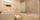How many CZK do we pay for lining the perimeter walls of the bathroom with rectangular shape with dimensions 3.5 m and 4 m, high 1.5 m if 1 square m tile cost 300 CZK?
• Money split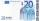Anton and Ferko got 2,500 euros together. The amount was split in a 1:4 ratio. How much did each get?
• 3 goods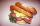Ms. Beránková bought in the shop 3 goods. Total paid for the purchase was 248 CZK. The first thing was 112 CZK and second 101 CZK. How much was the third thing?
• Square glassWhat is the area of the square window glass with the side 4 dm? Do you know how many cm2 is it?